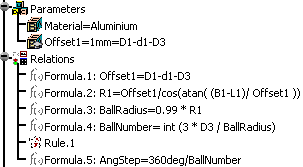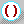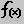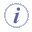Formulas are features used to define or constrain a parameter. A formula is a relation: the left part of the relation is the parameter to be constrained, the right part is a statement. After creating a formula, you can manipulate it like any other feature from its contextual menu. The formula language uses operators and functions of all types whereby you can perform operations on parameters.

## Displaying Formulas in the Specification Tree

Formulas are relations and as such they can be displayed below the Relations node provided you check the 'Relations' box below the 'Specification tree' settings in the Tools>Options>Infrastructure> Part Infrastructure>Display dialog box.

In addition, formulas can also be displayed below the Parameters node provided you check:

 the Parameters box below the Specification tree settings in the Tools>Options>Infrastructure> Part Infrastructure>Display  dialog box as well as the With Formula box below the Parameter Tree View settings in the  Tools>Options>General>Parameters and Measure dialog box## The Activity Parameter

A formula is a feature which is assigned a parameter called the activity. The activity value is a boolean. If the activity is set to true, the parameter value cannot be calculated from the formula. If a formula is created for a parameter which is not already constrained by another formula, the activity of the new formula is set to true by default.

A parameter can be constrained by several formulas, but only one formula can be active at a time. Before activating a formula on a given parameter, you must deactivate the other formulas defined on the same parameter.

 Activity value false true Relation icon in the specification tree## Importing Formulas

Parameters as well as the associated formulas can be imported from an external file. See Introducing Parameters and Importing Parameters for more information on how to import formulas.In the Formula editor, enumerations values are nls values. For example, when working with booleans, `true` and `false` will be displayed in your environment language.